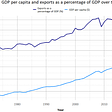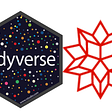# Part 1 : Preprocessing

This is part 1 of the tutorial series in which I introduce geoscientific data processing workflows using R. I used data downloaded from BCOGC Data Centre to investigate hydrocarbon production in British Columbia.

This is Part 1 of the tutorial in which I present how to load, merge and preprocess the original datasets and is only a subset of the whole analysis. The complete code including further steps as well as input data used can be found here.

Let’s get started.

`library(dplyr) # dataframe manipulation (e.g. renaming columns)                     library(stringr) # string manipulation`

We can turn off scientific notation on charts by using following code:

`options(scipen = 999) `

Let’s set the current working directory first, for example:

`path <- file.path("C:","Users","Desktop","R_Programming","RLadies_workshop")setwd(path)`

… and check if it worked using:

`sprintf("Current directory: %s", getwd())`

Now we can load some data from the zipped file:

`wells <- read.csv(unzip("BCOGC_data/prod_csv.zip", "zone_prd_2016_to_present.csv"), skip = 1) head(wells, 3)`

We can see that multiple columns have a lot of unnecessary dots. Let’s fix this using str_replace_all():

`names(wells) <- str_replace_all(names(wells), c("\\.."="_",                                                "\\."=""                                                ))`

and check the updated column names:

`head(wells)`

We are almost there, but still have 2 more things to fix:

1. select only relevant columns from the dataframe;
2. Find out which areas an formations correspond to the enigmatic codes, (which are not very informative).

Let’s use subset() to select only columns:

`wells <- subset(wells, select=c(Wa_num, Prod_period, Prod_days, Area_code, Formtn_code, Gas_prod_vol_e3m3, Oil_prod_vol_m3, Water_prod_vol_m3, Cond_prod_vol_m3))`

That correspond to well authorization number, production period, production days, area code, formation code, gas, oil, condensate and water production volume. Note the volumes are expressed in different units, cubic meters (m3) and thousand cubic meters (e3m3) depending on the production type.

Let’s take a look on two specific columns in our dataset: Area_code and Formation_code.

`head(subset(wells, select=c(Area_code, Formtn_code)), 3)`

Although they include relevant information about which area and formation was associated with given production wells, the numerical values are not so useful and we will retrieve the actual names from the ogc_area_codes.csv and ogc_formation_codes.csv files.

`area_codes <- read.table("BCOGC_data/ogc_area_codes.csv",                          sep = ",", skip = 1, header = TRUE, stringsAsFactors = FALSE)`

Here we used the comma as separator, skipping first row and using the column names from the .csv file as header. We also specify stringAsFactors = FALSE since we want our strings to stay strings (I won’t explain the difference between string and factor here, but I encourage you to google if you want to learn more).

`head(area_codes, 3)`
`area_codes <- rename(area_codes, Area_code = Area.Code, Area_name = Area.Name)`

Again, let’s rename the column Area.Name to Area_name to be consistent with wells dataframe (merge operation works only if column names are identical).

Let’s do the same for the second file…

`formation_codes <- read.table("BCOGC_data/ogc_formation_codes.csv",                               sep = ",", skip = 1, header = TRUE, stringsAsFactors = FALSE)formation_codes <- rename(formation_codes, Formtn_code = Formation.Code, Formtn_name = Formation.Name)`

…and check updated dataframes:

`head(area_codes,3)head(formation_codes,3)`

Now we can merge both dataframes with wells dataframe

`wells <- merge(wells, area_codes, by = 'Area_code')wells <- merge(wells, formation_codes, by = 'Formtn_code')`

Now let’s remove the “draft” dataframes from the memory and remove columns with area and formation codes (“-c” means subset everything except Area_code and Formtn_code).

`rm(area_codes, formation_codes) # remove form memorywells <- subset(wells, select = -c(Area_code, Formtn_code)) # remove from dataframehead(wells, 3)`

Once our dataset is ready, we can get some general information about our data.

We can use str() function to investigate types of variables in each column (integer, numeric or character) and example values:

`str(wells)`

We can also use summary() function to get some summary statistics in case of numeric variables and number of rows in case of character variables:

One thing which is particularly interesting is the number of formations in our dataset by calculating the length of the list consisting of unique values of Formtn_name column:

`length(unique(wells\$Formtn_name))`

We can also simply list them:

`unique(wells\$Formtn_name)`

Our dataset is ready! In the next part I will show how tidyr and dyplyr packages can be used to manipulate it further.

You can also check the complete code including further steps and input data on my github page.

--

--

--

Love podcasts or audiobooks? Learn on the go with our new app.

## VS Code Is Getting Much Better!## Blueberry world #7## Be Still in Her Presence## Change Data Capture using DMS## CS 373 Spring 2021 Week 3: Chanakya Remani## Gran Turismo 7: Update 1.06 With many improvements is available for download## R Language for The Project Management Course — AUG University “part 2”## Simple Guide: Changing fonts in ggplot2 with extrafont## Data Analysis with R — Part 3 (Control Flow)## Get your questions answered in minutes with R and Wolfram Alpha